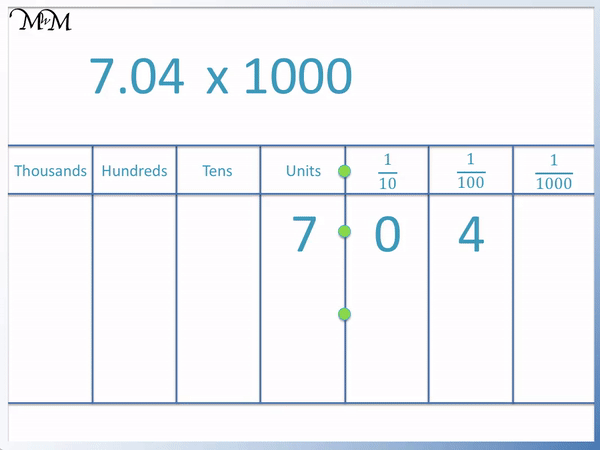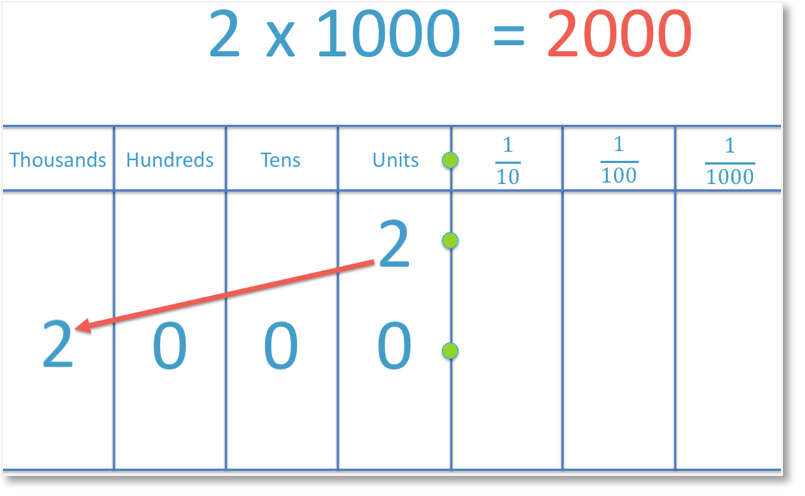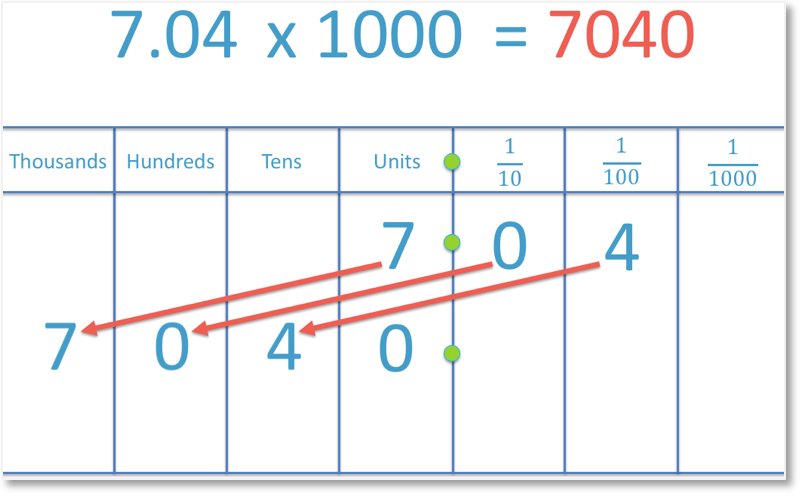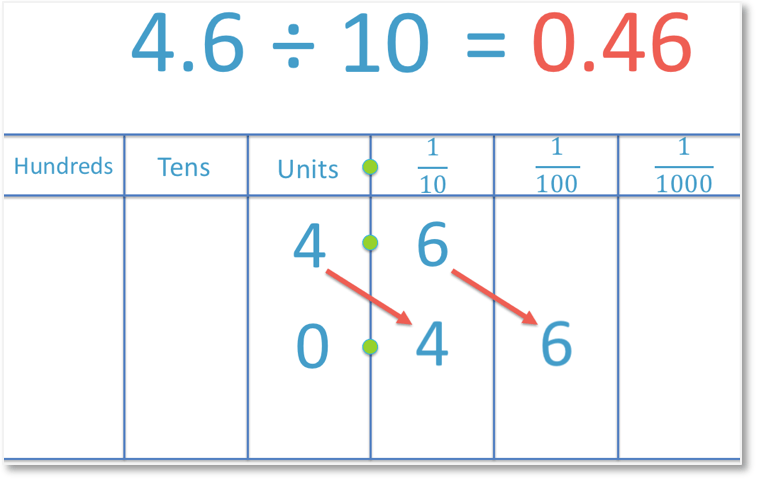# Multiplying by 1000

Multiplying by 1000• To multiply a number by one thousand, move each digit three place value columns to the left.
• To multiply 2 by 1000, the digit ‘2’ is moved three places to the left.
• ‘2’ moves from the units column into the thousands column.
• There are no digits in the hundreds, tens or units columns and so, a zero is written in each of these columns.
• 2 x 1000 = 2000.
• Multiplying a whole number by one thousand is the same as adding three zero digits to the end of this number.
To multiply a number by 1000, move all of its digits three places to the left.

Multiplying a whole number by 1000 is the same as adding three zeros to the end of the number.• To multiply a number by 1000, all digits of this number move three place value columns to the left.
• We will multiply the decimal number 7.04 by 1000.
• The digit ‘7’ moves from the units column to the thousands column.
• The digit ‘0’ moves from the tenths column to the hundreds column.
• The digit ‘4’ moves from the hundredths column to the tens column.
• There are no digits in the units column between the digit of ‘4’ and the decimal point and so a ‘0’ is put in its place.
• 7.04 x 1000 = 7040.Supporting Lessons# How do we Multiply a Number by 1000?

To multiply a number by 1000, move each digit in that number three place value columns to the left.

All digits in the original number remain in the same order.

To multiply a whole number by one thousand, a simple trick is to add three zero digits to the end of that whole number.

Here is an example of multiplying a whole number by 1000.In the example above, the 2 has been moved three places to the left. It has moved from the units column to the thousands column.

As there are no longer any digits in either the hundreds column, the tens column or the units column, we write a zero in each space to show that they are worth zero.

You may have already found that 2 x 1000 = 2000 by “adding three zeros”. This only works when multiplying whole numbers.

When teaching multiplying by 1000, it is best to show the digits moving three place value columns first. This is what is actually happening because 1000 is made of 10 x 10 x 10, which is the same as multiplying by 10 three times.

Each time we multiply by ten we move our digit one place value column to the left because our place value columns are in base ten and represent a multiplication by ten.

Most people will still ‘add three zeros’ when multiplying a whole number by 1000 and this is the easiest and best way to multiply a whole number by 1000 as it can be done quickly and mentally.

This trick should be shown after showing the digits moving in their place value columns and it should be emphasised that this only works with whole numbers.

It is important to understand how multiplying by 1000 works because not all numbers that we will be multiplying will be whole numbers.

Here is an example of multiplying a decimal number by 1000:To multiply the decimal number 0.05 by 1000, we move the 5 three places to the left. We move it from the hundredths column to the tens column. We must make sure that we show that the units column is worth zero by writing a zero in this space.

If there are no digits to the left of the decimal point, we always write a single zero digit in front of the decimal point to show this.

When multiplying a number with more than one digit by 1000, we need to make sure that we move every digit three places to the left.

For example:We begin by moving the 3 three places to the left. We move it from the tenths column to the hundreds column. The 9 can then follow. We move the 9 from the hundredths column to the tens column.

We must make sure that we show that the units column is worth zero by writing a zero in this space.

And so, 0.39 x 1000 = 390.

We can see the the digits in the original number, which are ‘3’ and ‘9’, remain in the same order in the answer and that they are still next to each other.

We need to be more careful when dealing with numbers where there is a zero between two other digits.

For example:We must make sure that we move every digit in 7.04 three places to the left, including the zero. We move the 7 from the units column to the thousands column, we move the 0 from the tenths column to the hundreds column and we move the 4 from the hundredths column to the tens column.

Because there are no longer any digits in the units column, we must write in a zero to show that it is worth zero.

The digits in the answer are in the same order as the digits in the original question. We have a ‘7’, followed by a ‘0’, followed by a ‘4’.

We can us this to help us multiply numbers by 1000 quickly and mentally.

We know that when we are multiplying by 1000 any digits in the units column will move to the thousands column.

We have a ‘7’ in the units column, so we know that when we multiply by 1000, the answer will be 7 thousand and something.

We simply move the 7 to the thousands column and repeat the following digits in that same order. So we put the ‘0’ and the ‘4’ immediately afterwards.

When teaching multiplication by 1000, we can start by showing the place value columns and with practice, knowing that the digits will remain in the same order, we can speed this up to doing it mentally.Now try our lesson on Dividing by 10 where we learn how to divide a number by 10.error: Content is protected !!